# 认识Arduino与C语言的指针和指针数组

#### 建立函式指针程序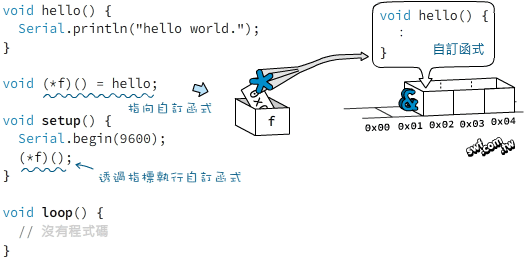#### 函式指针的语法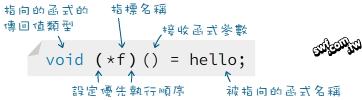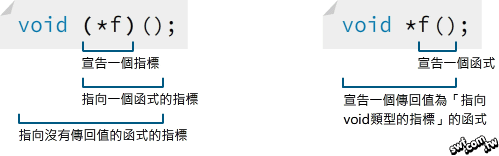```#include <stdio .h>

// 宣告自訂函式
void hello() {
printf("hello world.\n");
}

// 宣告指向hello函式的函式指標
void (*f)() = hello;

void main(void) {
// 透過函式指標執行hello函式
(*f)();
}
</stdio>
```

#### 函式指针的参数传递与传回值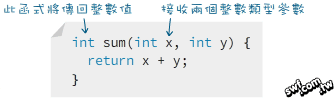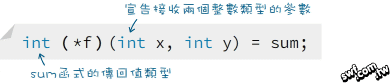```// 宣告自訂函式
int sum(int x, int y) {
return x + y;
}

// 宣告具有兩個參數以及整數傳回值的函式指標
int (*f)(int x, int y) = sum;

void setup() {
Serial.begin(9600);

// 執行函式指標並傳遞兩個參數
int val = (*f)(8, 4);

// 將在序列埠監控視窗顯示"Ans: 12"
Serial.print("Ans: ");
Serial.println(val);
}

void loop() {
// 這裡沒有程式
}
```

```#include <stdio .h>

int sum(int x, int y) {
return x + y;
}

int (*f)(int x, int y) = sum;

void main(void) {
int val = (*f)(8, 4);

printf("Ans: %d\n", val);
}
</stdio>
```

#### 函式指针数组```// 宣告三個自訂函式
void fn0() {
Serial.println("Hello from fn0.");
}

void fn1() {
Serial.println("Hello from fn1.");
}

void fn2() {
Serial.println("Hello from fn2.");
}

// 建立函式陣列指標
void (*f) () = {fn0,fn1,fn2};

void setup() {
Serial.begin(9600);
Serial.println("Please input number 0~2:");
}

void loop() {
if( Serial.available() ) {
// 讀取序列輸入值，並轉換成整數。
byte val = Serial.read() - 48;
// 確認輸入值介於0和2之間
if (val >= 0 && val < = 2) {
// 將val當作陣列索引，執行對應的函式。
(*f[val])();
} else {
// 若輸入值超過指定範圍，則顯示“Wrong number!”（錯誤數字！）
Serial.println("Wrong number!");
}
}
}
```

```#include <stdio .h>

void fn0() {
printf("Hello from fn0.\n");
}

void fn1() {
printf("Hello from fn1.\n");
}

void fn2() {
printf("Hello from fn2.\n");
}

void (*f) () = {fn0,fn1,fn2};

void main(void) {
int val;

printf("\nPlease input number 0~2:");
// 讀取輸入值
scanf("%d",&val);

if(val>=0 && val< =2) {
(*f[val])();
} else {
printf("Wrong number!\n");
}
}
```